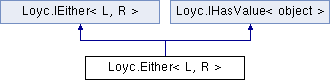Enhanced C# Language of your choice: library documentation
Loyc.Either< L, R > Struct Template Reference

Holds a single value of one of two types (L or R). More...

Source file:
Inheritance diagram for Loyc.Either< L, R >:## Remarks

Holds a single value of one of two types (L or R).

For efficiency, this is a struct, but this makes it possible to default-construct it. In that case its value will be `default(R)`.

## Public fields

Maybe< L > Left => _hasLeft ? _left : new Maybe<L>()

Maybe< R > Right => _hasLeft ? new Maybe<R>() : _right

object Value => _hasLeft ? (object)_left : _right

IMaybe< L > IEither< L, R >. Left => Left

IMaybe< R > IEither< L, R >. Right => Right

## Public Member Functions

static Either< L, R > R (value)

static Either< L, R > R (value)

Either (L value)

Either (R value)

Either< L2, R2 > Select< L2, R2 > (Func< L, L2 > selectL, Func< R, R2 > selectR)
Converts an Either to another with different types. More...

Either< L2, R2 > R2(selectL(_left)) Either< L2, R > MapLeft< L2 > (Func< L, L2 > selectL)
Transforms `Left` with the given selector, if `Left.HasValue`. Otherwise, returns Right unchanged. More...

Either< L2, R2 > R2(selectL(_left)) Either< L2, R > R(selectL(_left)) Either< L, R2 > MapRight< R2 > (Func< R, R2 > selectR)
Transforms `Right` with the given selector, if `Right.HasValue`. Otherwise, returns Left unchanged. More...

Either< L2, R2 > R2(selectL(_left)) Either< L2, R > R(selectL(_left)) Either< L, R2 > R2(selectR(_right)) Either< L, R > IfLeft (Action< L > actionL)
Runs actionL if `Left.HasValue`. Equivalent to `Left.Then(actionL)`, but also returns `this`. More...

Either< L, R > IfRight (Action< R > actionR)
Runs actionR if `Right.HasValue`. Equivalent to `Right.Then(actionL)`, but also returns `this`. More...

## Static Public Member Functions

static Either< L, R > NewLeft (L value)
Simply calls the constructor. This method exists to make it possible to construct an Either when both types are the same. More...

static Either< L, R > NewRight (R value)
Simply calls the constructor. This method exists to make it possible to construct an Either when both types are the same. More...

static implicit operator Either< L, R > (L value)

static implicit operator Either< L, R > (R value)

static Either< L, R > From< L2, R2 > (Either< L2, R2 > x)
Does an upcast, e.g. Either{string,ArgumentException} to Either{object,Exception}. C# does not allow defining conversion operators to take generic parameters, so you'll have to put up with this hassle instead. More...Properties inherited from Loyc.IEither< L, R >
IMaybe< L > Left` [get]`

IMaybe< R > Right` [get]`Properties inherited from Loyc.IHasValue< object >
Value` [get]`

## ◆ From< L2, R2 >()

 static Either Loyc.Either< L, R >.From< L2, R2 > ( Either< L2, R2 > x )
static

Does an upcast, e.g. Either{string,ArgumentException} to Either{object,Exception}. C# does not allow defining conversion operators to take generic parameters, so you'll have to put up with this hassle instead.

Sadly, automatically upcasting value types to reference types doesn't seem possible.

Type Constraints
 L2 : L R2 : R R2 : new R2 : Either R2 : L R2 : R R2 : x._hasLeft R2 : x._left R2 : x._right

## ◆ IfLeft()

 Either R2 (selectL(_left)) Either R (selectL(_left)) Either R2 (selectR(_right)) Either Loyc.Either< L, R >.IfLeft ( Action< L > actionL )
inline

Runs actionL if `Left.HasValue`. Equivalent to `Left.Then(actionL)`, but also returns `this`.

## ◆ IfRight()

 Either Loyc.Either< L, R >.IfRight ( Action< R > actionR )
inline

Runs actionR if `Right.HasValue`. Equivalent to `Right.Then(actionL)`, but also returns `this`.

## ◆ MapLeft< L2 >()

 Either R2 (selectL(_left)) Either Loyc.Either< L, R >.MapLeft< L2 > ( Func< L, L2 > selectL )

Transforms `Left` with the given selector, if `Left.HasValue`. Otherwise, returns Right unchanged.

## ◆ MapRight< R2 >()

 Either R2 (selectL(_left)) Either R (selectL(_left)) Either Loyc.Either< L, R >.MapRight< R2 > ( Func< R, R2 > selectR )

Transforms `Right` with the given selector, if `Right.HasValue`. Otherwise, returns Left unchanged.

## ◆ NewLeft()

 static Either Loyc.Either< L, R >.NewLeft ( L value )
static

Simply calls the constructor. This method exists to make it possible to construct an Either when both types are the same.

## ◆ NewRight()

 static Either Loyc.Either< L, R >.NewRight ( R value )
static

Simply calls the constructor. This method exists to make it possible to construct an Either when both types are the same.

## ◆ Select< L2, R2 >()

 Either Loyc.Either< L, R >.Select< L2, R2 > ( Func< L, L2 > selectL, Func< R, R2 > selectR )

Converts an Either to another with different types.